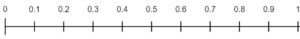# Decimals on a Number Line Worksheets

• ###### Decimals on a Number Line #15

Decimals on a Number Line worksheets are an instructional tool used to help students visualize and understand the concept of decimal numbers and their relative positions to one another. They use a number line, a visual representation of numbers placed in order on a horizontal line, to graphically demonstrate where decimal numbers fit in relation to whole numbers and other decimal numbers.

These worksheets contain several types of exercises aimed at teaching students how to work with decimal numbers using number lines. Here are some common types of exercises:

Plotting Decimals – The most fundamental exercise, this requires students to mark or plot a given decimal on the number line. For instance, a student might be given the number 0.5 and asked to place it on the number line.

Identifying Decimals – These exercises present a number line with certain points already marked. The student’s task is to identify which decimal number corresponds to each marked point.

Comparing Decimals – Here, students are given two or more decimal numbers and asked to plot them on the number line. This visual representation helps students understand which decimal is greater or lesser.

Ordering Decimals – In these exercises, students are given multiple decimal numbers to plot on the number line, helping them understand the correct ascending or descending order.

Estimating Decimals – These exercises involve estimating the value of a point on the number line that isn’t labeled by reasoning about the positions of numbers.

Word Problems – Real-world scenarios that require the use of the number line to solve problems involving decimals.

These exercises are incredibly beneficial for enhancing a student’s overall math skills:

Understanding Decimal Numbers – The visual representation of decimals on a number line provides students with a clearer concept of what decimal numbers represent and how they relate to whole numbers and each other.

Enhancing Number Sense – By visually comparing and ordering decimal numbers, students develop a stronger understanding of the number system and how numbers relate to one another.

Developing Estimation Skills – When students estimate where a decimal should be placed on a number line, they are honing their ability to make mathematical estimations.

Building Problem-Solving Skills – Word problems require students to apply what they’ve learned about decimals on a number line to solve real-world problems.

The skills gained from using Decimals on a Number Line worksheets are applicable in various real-life scenarios. For instance, understanding decimals can help when dealing with money, as most currencies are divided into a decimal system with 100 smaller units making up the main unit. In measurements too, for instance, when determining distances or quantities in recipes, understanding decimals is crucial. The number line representation also develops a visual understanding of math, which can be helpful in many professions like engineering, architecture, data analysis, and more.

### How Do You Plot a Decimal Value on a Number Line?

Plotting a decimal value on a number line can be a great way to visualize the value of the number and its relation to other numbers. Here’s a step-by-step guide on how to do it:

Draw a Number Line – Start by drawing a horizontal line. This represents your number line.

Mark the Number Line – Decide on the range of numbers that your number line will represent. For example, if you are plotting decimals between 0 and 1, you would place a small mark or line at each end of your number line to represent these numbers. Write “0” below the line on the left side and “1” below the line on the right side.

Divide the Number Line – Divide the space between 0 and 1 into 10 equal parts, each part represents one-tenth (0.1). This is the typical setup when dealing with decimals, but you could divide into more sections if you’re dealing with hundredths (0.01), thousandths (0.001), and so forth.Identify the Decimal – Identify the decimal number that you are trying to plot. Let’s say the number is 0.4.

Plot the Decimal – To plot a decimal like 0.4, count four-tenths (or four of the equal parts you divided your line into) from 0 and make a mark. That’s where 0.4 would be located on the number line.

Label the Point – Finally, label the point with the decimal number, in this case, 0.4.

Remember, the steps may vary slightly depending on the decimal you’re plotting. If you’re plotting a number like 1.4, your number line would need to extend from 0 to at least 2 and then you’d count one whole and four-tenths to find where to plot your decimal.

Using a number line to understand decimals is an excellent way to visualize them, which can be especially helpful when learning about these types of numbers for the first time. It’s also a useful tool when it comes to comparing decimals or when you’re learning to round decimals.